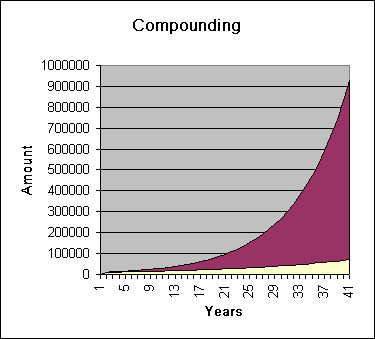Here’s a useful tool to calculate the cost of Usury on a loan based on annuities: regular equal payments over a period. It for instance calculates the cost of Usury on a mortgage at any given principal/interest rate/period.

It’s a simple excel sheet that allows you to change Principal, number of payments and Interest Rates.

It can be found permanently at the Resource Page.

Here goes: annuity

1.•